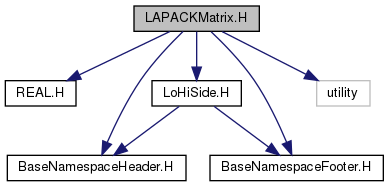Chombo + EB  3.2
LAPACKMatrix.H File Reference
`#include "REAL.H"`
`#include "LoHiSide.H"`
`#include <utility>`
`#include "BaseNamespaceHeader.H"`
`#include "BaseNamespaceFooter.H"`
Include dependency graph for LAPACKMatrix.H:Go to the source code of this file.

## Classes

class  LAPACKMatrix

## Macros

#define _LAPACKMATRIX_H_

## Functions

Real getInverseOfConditionNumber (const LAPACKMatrix &A)

void multiply (LAPACKMatrix &a_product, const LAPACKMatrix &a_left, const LAPACKMatrix &a_right)

int solveLeastSquares (LAPACKMatrix &A, LAPACKMatrix &B)
below stuff is shamelessly stolen from lapackwrapper class More...

int solveLeastSquaresTranspose (LAPACKMatrix &A, LAPACKMatrix &B)

int solveLSTSVDOnce (LAPACKMatrix &X, const LAPACKMatrix &A, const LAPACKMatrix &B)

int solveLSTSVD (LAPACKMatrix &A, LAPACKMatrix &B, int a_maxiter, Real a_tol)

int solveEqualityConstrainedLS (LAPACKMatrix &A, LAPACKMatrix &c, LAPACKMatrix &B, LAPACKMatrix &d, LAPACKMatrix &x)

int solveReducedRankLS (LAPACKMatrix &A, LAPACKMatrix &b)

## ◆ _LAPACKMATRIX_H_

 #define _LAPACKMATRIX_H_

## ◆ getInverseOfConditionNumber()

 Real getInverseOfConditionNumber ( const LAPACKMatrix & A )

Following Lapack, gets inverse of condition number. Returning a number near zero means the matrix is not really solvable.

## ◆ multiply()

 void multiply ( LAPACKMatrix & a_product, const LAPACKMatrix & a_left, const LAPACKMatrix & a_right )

sets product = a_left* a_right fails if a_left.m_col != a_right.m_rows

## ◆ solveLeastSquares()

 int solveLeastSquares ( LAPACKMatrix & A, LAPACKMatrix & B )

below stuff is shamelessly stolen from lapackwrapper class

Solves A*X = B using general least squares, for each column of B

## ◆ solveLeastSquaresTranspose()

 int solveLeastSquaresTranspose ( LAPACKMatrix & A, LAPACKMatrix & B )

Solves A'*X = B using least squares, for vector b

## ◆ solveLSTSVDOnce()

 int solveLSTSVDOnce ( LAPACKMatrix & X, const LAPACKMatrix & A, const LAPACKMatrix & B )

Solves A*X = B using least squares with SVD, for X

## ◆ solveLSTSVD()

 int solveLSTSVD ( LAPACKMatrix & A, LAPACKMatrix & B, int a_maxiter, Real a_tol )

Solves A^T X = B using least squares with SVD, for vector b

## ◆ solveEqualityConstrainedLS()

 int solveEqualityConstrainedLS ( LAPACKMatrix & A, LAPACKMatrix & c, LAPACKMatrix & B, LAPACKMatrix & d, LAPACKMatrix & x )

Solves equality constrained least squares problem Find x, s.t. min norm(A x - c) with B x = d

## ◆ solveReducedRankLS()

 int solveReducedRankLS ( LAPACKMatrix & A, LAPACKMatrix & b )

Solves A'*X = B using reduced rank least squares, for vector b